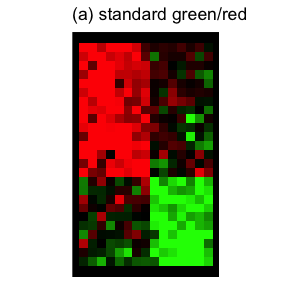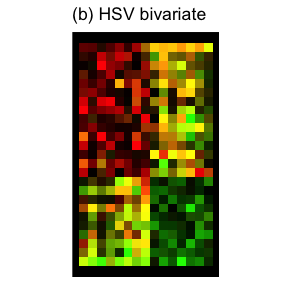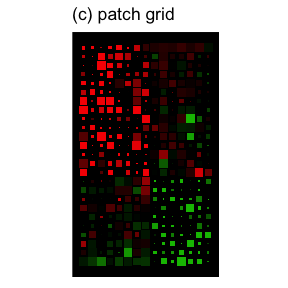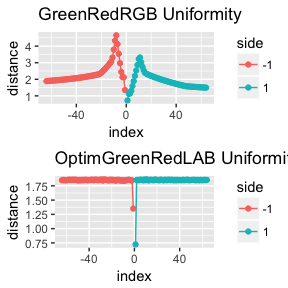# colorpatch Package Introduction

#### 2017-06-09

An important step in analyzing high dimensional data is the inspection of visual maps before the application of automatic analysis techniques. Here we present a method for visualizing fold changes and confidence values within a single diagram. Fold changes (or ratios) naturally occur when comparing a measurement value $$B$$ with a control condition $$A > 0$$ as it occurs in analyzing gene expression, agricultural, or financial data. Usually fold changes $$r$$ are defined by: $r = \frac{B - A}{A}$ or (especially in gene expressions): $r = \log_2\frac{B}{A}$ High dimensional data such as gene expression profiles of different conditions are traditionally visualized as a patch grid showing fold changes (in this case the log ratios) of different genes and multiple samples. The inspection of these maps is known to be prone to errors, if no other information than the fold changes is taken into account (Bilban et al. 2002). The absolute (logarithmic) intensities can be seen as a confidence measure for the observed ratios: $a = \frac{1}{2} log_2{(A\cdot B)} \qquad A > 0, B > 0$ Other possibilities for computing confidence values may include statistical models.

The colorpatch package introduces a new bi-variate patch grid visualization for showing fold changes $$r_{ij}$$ of different samples $$j=1\ldots m$$ among multiple conditions $$i=1\ldots n$$ (e.g. genes) together with confidence values $$a_{ij}$$ within a single visual map. A psychophysically optimized palette [colorpatch::OptimGreenRedLAB] is used with this visualization scheme for an optimal visual performance.

The package also contains the code for the optimization of bi-colored color palettes (see Kestler et al. 2006). As the generation of these palettes is time consuming in the R some of them are pre-computed in the data directory (use the data() function for loading these palettes):

• GreenRedRGB - linearly scales the green channel and the red channel
• OptimGreenRedLAB - perceptually optimized green/red palette in the LAB color space
• OptimBlueYellowLAB - perceptually optimized blue/yellow palette in the LAB color space

Re-generation of the palettes can be performed with the following call:

    GeneratePalettes()

## The Patch Grid Approach

The colorpatch package provides color grids of different types:

1. Standard green/red mappings of fold changes.
2. Bivariate color maps (e.g. HSV) showing fold changes and confidence values encoded as a single color.
3. Patch grids showing fold changes encoded as color and confidence values encoded as patch sizes.

### Example Data Set

In the following a random data set is generated

dat <- CreateClusteredData(ncol.clusters = 3, nrow.clusters = 3,
nrow = 25, ncol = 15, alpha = 50)

ordered, and pre-processed into a data-frame:

dat <- OrderData(dat)
df <- ToDataFrame(dat)

## Comparing the Visualization Approaches

All three approaches are used to visualize the same data set. Cutoff values for fold changes (ratios) and confidence values are set to $$0.5$$:

thresh.ratio <- 0.5 * max(abs(dat$ratio)) thresh.conf <- 0.5 * max(dat$conf)

For rendering the data the colorpatch package extends the ggplot2 package with two new statistics stat_colorpatch and stat_bicolor:

p <- ggplot(df, aes(ratio = ratio, conf = conf, x = x, y = y))
p <- p + theme_colorpatch(plot.background = "white") + coord_fixed(ratio = 1)

p + stat_colorpatch(aes(ratio = ratio, conf = 1, x = x, y = y),
thresh.ratio = thresh.ratio,
color.fun = ColorPatchColorFun("GreenRedRGB")) +
ggtitle("(a) standard green/red")

p + stat_bicolor(thresh.ratio = thresh.ratio,
thresh.conf = thresh.conf) +
ggtitle("(b) HSV bivariate")

p + stat_colorpatch(thresh.ratio = thresh.ratio,
thresh.conf = thresh.conf) +
ggtitle("(c) patch grid")# Comparing the Perceptual Uniformity of the Palettes

In the following the uniformity within the LAB color space for the standard RGB palette and the OptimGreenRedLAB palettes are displayed.

data("GreenRedRGB")
data("OptimGreenRedLAB")
grid.newpage()
pushViewport(viewport(layout = grid.layout(2, 1),
gp = gpar(fill = "black", col = "black", lwd = 0)))
p0 <- PlotUniformity(GreenRedRGB) + ggtitle("GreenRedRGB Uniformity")
p1 <- PlotUniformity(OptimGreenRedLAB) + ggtitle("OptimGreenRedLAB Uniformity")
print(p0, vp = vplayout(1, 1))
print(p1, vp = vplayout(2, 1))
popViewport()Comparing the uniformity of standard RGB and OPT palette. The Euclidean distances within the LAB colorspace between adjacent colors are shown.

# Bibliograhpy

Bilban, M, LK Buehler, S Head, G Desoye, and V Quaranta. 2002. “Defining Signal Thresholds in Dna Microarrays: Exemplary Application for Invasive Cancer.” BMC Genomics 3 (1). BioMed Central: 1.

Kestler, Hans A., André Müller, Malte Buchholz, Thomas M. Gress, and Günther Palm. 2006. “A Perceptually Optimized Scheme for Visualizing Gene Expression Ratios with Confidence Values.” In Perception and Interactive Technologies, edited by E. André, L. Dybkjær, W. Minker, H. Neumann, and M. Weber, 4021:73–84. LNAI. Berlin: Springer.